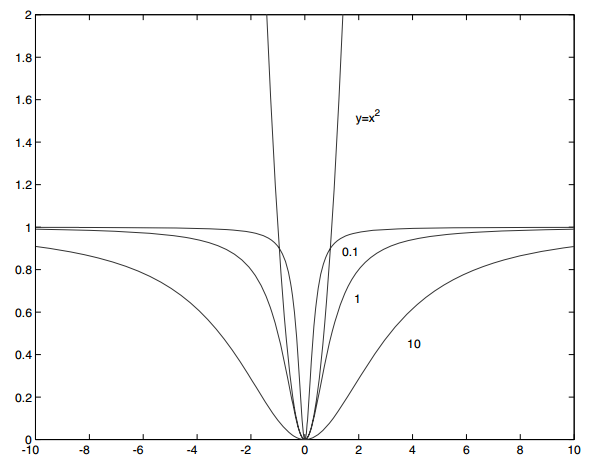## 理解图像配准中的LMeds、M-estimators与RANSAC算法

2015年10月15日

### 最小中值法（LMedS）

LMedS的做法很简单，就是从样本中随机抽出N个样本子集，使用最大似然（通常是最小二乘）对每个子集计算模型参数和该模型的偏差，记录该模型参数及子集中所有样本中偏差居中的那个样本的偏差（即Med偏差），最后选取N个样本子集中Med偏差最小的所对应的模型参数作为我们要估计的模型参数。

$$\theta=arg_{\theta}\min med_{i \in N}r_{i}(x_{i},\theta)$$

$\theta$为拟合模型参数，$r_{i}(x_{i},\theta)$是模型第i个点的残留误差，N是我们随机抽出的样本子集，$med$为中值操作。

### M估计（M-estimators）

$$\theta=arg_{\theta} \sum_{i}\rho(r_{i}(x_{i},\theta);\sigma)$$

$$\rho(\mu,\sigma)=\frac{\mu^{2}}{\sigma^{2}+\mu^{2}}$$

$\sigma$参数是控制函数变平点的变量，下图是当$\sigma^{2}=0.1, 1, 10$时画的$rho(x,\sigma)=\frac{x^{2}}{\sigma^{2}+x^{2}}$均匀随机抽取$r$个不同点组成样本子集

用最大似然（通常是最小二乘）对抽取的点集进行拟合获得$\theta_{s}^{0}$

用$\theta_{s}^{n-1}$估计$\sigma_{s}^{n-1}$，一个流行的估计式是：$\sigma^{n-1}=1.4826median_{i}|r_{i}^{n-1};\theta^{n-1}|$

直到收敛（$|\theta_{s}^{n}-\theta_{s}^{n-1}|$非常小）

用最小化方法通过$\theta_{s}^{n-1},\sigma_{s}^{n-1}$得到$\theta_{s}^{n}$

计算$\sigma_{s}^{n}$

End

End

### 随机采样一致算法（RANSAC）

M估计计算复杂性较高。另外一种最常用的方法是随机采样一致算法（RANSAC，Random Sample Consensus）。它的基本思路是随机选择一个小的数据点子集，然后对其进行拟合，查看有多少其他点匹配到拟合的模型上，迭代这个过程直至有较大的概率找到我们想要拟合的模型。

$n$——所需要的最少点数

$k$——需要的迭代次数

$d$——判断一个点是否拟合的很好的阈值，也称内外点距离阈值

$t$——判断一个模型是否拟合的很好所需要的邻近点数目，也称一致性集合大小阈值

从数据中均匀的采样$n$个点

对这$n$个点进行模型拟合

对于采样外的每个点

用$d$比较点到拟合模型的距离，如果距离小于$d$，则认为点是靠近的

End

如果有$t$个或者更多的点靠近模型，则认为该拟合是个好的拟合，重新用这些点和靠近的点拟合模型。

End

RANSAC的难点是$n,k,d,t$这几个参数的确定，下面就怎么确定参数做简单的讨论。

$$1-p=(1-w^{n})^{k} \Rightarrow k=\frac{log(1-p)}{log(1-w^{n})}$$

### 参考文献

 图像局部不变性特征与描述.王永明 王贵锦. 国防工业出版社.

 Computer Vision: A Modern Approach. David A. Forsyth and Jean Poince.